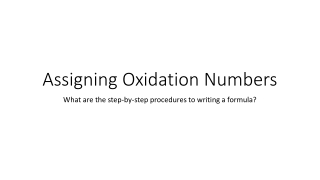DownloadDownload PresentationAssigning Oxidation Numbers

# Assigning Oxidation Numbers

Télécharger la présentation## Assigning Oxidation Numbers

- - - - - - - - - - - - - - - - - - - - - - - - - - - E N D - - - - - - - - - - - - - - - - - - - - - - - - - - -
##### Presentation Transcript

1. Assigning Oxidation Numbers What are the step-by-step procedures to writing a formula?

2. Did you notice anything during the do-now activity? • Were there any coincidences? Let’s talk about it.

3. Rules for assigning Oxidation numbers • The oxidation number of free elements is always zero (Example: Cl0, Mg0, H20) • This includes diatomic elements (H2 N2 F2 O2 I2 Cl2 Br2) and certain molecular substances (P4 and S8) • A good way to remember these elements is with the phrase "BrINClHOF"

4. Continued 2. The oxidation number of a monatomic ion is equal to its charge Example: Na+1 means that its oxidation number is +1 Cl-1 means that its oxidation number is -1

5. Continued 3. The algebraic sum of the oxidation numbers of all the atoms in the formula of a compound is zero Example: NaCl Na+1 Cl-1 1 + (-1)= 0 ***NOTE: for the most part, the first element will have a POSITIVE CHARGE and the second element will have a NEGATIVE CHARGE***

6. Continued 4. The oxidation number of hydrogen is +1 5. The oxidation number of oxygen is -2

7. Continued 6. The algebraic sum of the atoms in the formula of polyatomic ion (found on table E) is equal to its charge: Example: CN-1 C+2 N-3 +2 + (-3) = -1

8. Continued 7. In combinations of non-metals, the oxidation number of the less electronegative element is positive and that of the more electronegative element is negative. Example: HF: (H electronegativity= 2.2) (F electronegativity= 4.0) Because of this, hydrogen’s charge will be positive and fluorine’s charge will be negative H+1F-1

9. Let’s try some together: • Cu • F2 • H2O • MgI2 • CuSO4

10. Exceptions for assigning oxidation numbers • NH4+1 : when assigning the oxidation number for these, the only way this works is if nitrogen has a negative charge. • Example: N-3 H4+1 • (-3) + 1(4)= +1 • O2-2: to assign peroxide each element’s oxidation number, the only way it can add up to -2 is if each oxidation number is -1 • Example: O-1O-1 • (-1) + (-1) = -2

11. Continued 3. OH-1: The only way these oxidation numbers will work is if the first element (oxygen) in this compound has a negative charge: Example: O-2 H+1 -2 + 1= -1 4. S2O3-2: For this compound, the only way that it works is if Sulfur has a positive 2 charge: Example: S2+2 O3-2 2(2) + 3(-2) 4+(-6) =-2